Download Presentation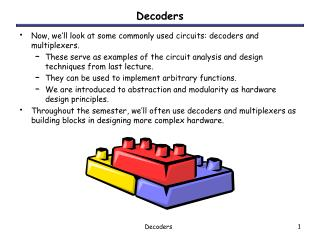Decoders

# Decoders - PowerPoint PPT Presentation

Decoders. Now, we’ll look at some commonly used circuits: decoders and multiplexers. These serve as examples of the circuit analysis and design techniques from last lecture. They can be used to implement arbitrary functions.I am the owner, or an agent authorized to act on behalf of the owner, of the copyrighted work described.
Download Presentation## Decoders

An Image/Link below is provided (as is) to download presentation

Download Policy: Content on the Website is provided to you AS IS for your information and personal use and may not be sold / licensed / shared on other websites without getting consent from its author.While downloading, if for some reason you are not able to download a presentation, the publisher may have deleted the file from their server.

- - - - - - - - - - - - - - - - - - - - - - - - - - E N D - - - - - - - - - - - - - - - - - - - - - - - - - -
Presentation Transcript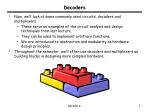Decoders
• Now, we’ll look at some commonly used circuits: decoders and multiplexers.
• These serve as examples of the circuit analysis and design techniques from last lecture.
• They can be used to implement arbitrary functions.
• We are introduced to abstraction and modularity as hardware design principles.
• Throughout the semester, we’ll often use decoders and multiplexers as building blocks in designing more complex hardware.

Decoders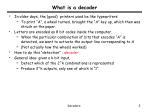What is a decoder
• In older days, the (good) printers used be like typewriters:
• To print “A”, a wheel turned, brought the “A” key up, which then was struck on the paper.
• Letters are encoded as 8 bit codes inside the computer.
• When the particular combination of bits that encodes “A” is detected, we want to activate the output line corresponding to A
• (Not actually how the wheels worked)
• How to do this “detection” : decoder
• General idea: given a k bit input,
• Detect which of the 2^k combinations is represented
• Produce 2^k outputs, only one of which is “1”.

Decoders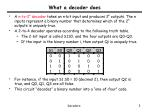What a decoder does
• A n-to-2ndecoder takes an n-bit input and produces 2n outputs. The n inputs represent a binary number that determines which of the 2n outputs is uniquely true.
• A 2-to-4 decoder operates according to the following truth table.
• The 2-bit input is called S1S0, and the four outputs are Q0-Q3.
• If the input is the binary number i, then output Qi is uniquely true.
• For instance, if the input S1 S0 = 10 (decimal 2), then output Q2 is true, and Q0, Q1, Q3 are all false.
• This circuit “decodes” a binary number into a “one-of-four” code.

Decoders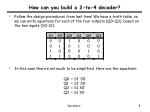How can you build a 2-to-4 decoder?
• Follow the design procedures from last time! We have a truth table, so we can write equations for each of the four outputs (Q0-Q3), based on the two inputs (S0-S1).
• In this case there’s not much to be simplified. Here are the equations:

Q0 = S1’ S0’

Q1 = S1’ S0

Q2 = S1 S0’

Q3 = S1 S0

Decoders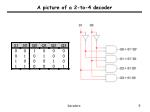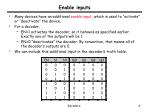Enable inputs
• Many devices have an additional enable input, which is used to “activate” or “deactivate” the device.
• For a decoder,
• EN=1 activates the decoder, so it behaves as specified earlier. Exactly one of the outputs will be 1.
• EN=0 “deactivates” the decoder. By convention, that means all of the decoder’s outputs are 0.
• We can include this additional input in the decoder’s truth table:

Decoders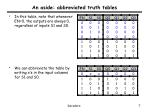An aside: abbreviated truth tables
• In this table, note that whenever EN=0, the outputs are always 0, regardless of inputs S1 and S0.
• We can abbreviate the table by writing x’s in the input columns for S1 and S0.

Decoders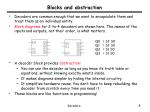Blocks and abstraction
• Decoders are common enough that we want to encapsulate them and treat them as an individual entity.
• Block diagrams for 2-to-4 decoders are shown here. The names of the inputs and outputs, not their order, is what matters.
• A decoder block provides abstraction:
• You can use the decoder as long as you know its truth table or equations, without knowing exactly what’s inside.
• It makes diagrams simpler by hiding the internal circuitry.
• It simplifies hardware reuse. You don’t have to keep rebuilding the decoder from scratch every time you need it.
• These blocks are like functions in programming!

Q0 = S1’ S0’

Q1 = S1’ S0

Q2 = S1 S0’

Q3 = S1 S0

Decoders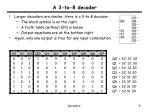A 3-to-8 decoder
• Larger decoders are similar. Here is a 3-to-8 decoder.
• The block symbol is on the right.
• A truth table (without EN) is below.
• Output equations are at the bottom right.
• Again, only one output is true for any input combination.

Q0 = S2’ S1’ S0’

Q1 = S2’ S1’ S0

Q2 = S2’ S1 S0’

Q3 = S2’ S1 S0

Q4 = S2 S1’ S0’

Q5 = S2 S1’ S0

Q6 = S2 S1 S0’

Q7 = S2 S1 S0

Decoders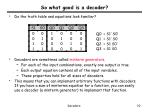So what good is a decoder?
• Do the truth table and equations look familiar?
• Decoders are sometimes called minterm generators.
• For each of the input combinations, exactly one output is true.
• Each output equation contains all of the input variables.
• These properties hold for all sizes of decoders.
• This means that you can implement arbitrary functions with decoders. If you have a sum of minterms equation for a function, you can easily use a decoder (a minterm generator) to implement that function.

Q0 = S1’ S0’

Q1 = S1’ S0

Q2 = S1 S0’

Q3 = S1 S0

Decoders• Let’s make a circuit that adds three 1-bit inputs X, Y and Z.
• We will need two bits to represent the total; let’s call them C and S, for “carry” and “sum.” Note that C and S are two separate functionsof the same inputs X, Y and Z.
• Here are a truth table and sum-of-minterms equations for C and S.

C(X,Y,Z) = m(3,5,6,7)

S(X,Y,Z) = m(1,2,4,7)

0 + 1 + 1 = 10

1 + 1 + 1 = 11

Decoders• Here, two 3-to-8 decoders implement C and S as sums of minterms.
• The “+5V” symbol (“5 volts”) is how you represent a constant 1 or true in LogicWorks. We use it here so the decoders are always active.

C(X,Y,Z) = m(3,5,6,7)

S(X,Y,Z) = m(1,2,4,7)

DecodersUsing just one decoder
• Since the two functions C and S both have the same inputs, we could use just one decoder instead of two.

C(X,Y,Z) = m(3,5,6,7)

S(X,Y,Z) = m(1,2,4,7)

DecodersBuilding a 3-to-8 decoder
• You could build a 3-to-8 decoder directly from the truth table and equations below, just like how we built the 2-to-4 decoder.
• Another way to design a decoder is to break it into smaller pieces.
• Notice some patterns in the table below:
• When S2 = 0, outputs Q0-Q3 are generated as in a 2-to-4 decoder.
• When S2 = 1, outputs Q4-Q7 are generated as in a 2-to-4 decoder.

Q0 = S2’ S1’ S0’ = m0

Q1 = S2’ S1’ S0 = m1

Q2 = S2’ S1 S0’ = m2

Q3 = S2’ S1 S0 = m3

Q4 = S2 S1’ S0’ = m4

Q5 = S2 S1’ S0 = m5

Q6 = S2 S1 S0’ = m6

Q7 = S2 S1 S0 = m7

DecodersDecoder expansion
• You can use enable inputs to string decoders together. Here’s a 3-to-8 decoder constructed from two 2-to-4 decoders:

DecodersModularity
• Be careful not to confuse the “inner” inputs and outputs of the 2-to-4 decoders with the “outer” inputs and outputs of the 3-to-8 decoder (which are in boldface).
• This is similar to having several functions in a program which all use a formal parameter “x”.
• You could verify that this circuit is a 3-to-8 decoder, by using equations for the 2-to-4 decoders to derive equations for the 3-to-8.

DecodersA variation of the standard decoder
• The decoders we’ve seen so far are active-high decoders.
• An active-low decoder is the same thing, but with an inverted EN input and inverted outputs.

DecodersSeparated at birth?
• Active-high decoders generate minterms, as we’ve already seen.
• The output equations for an active-low decoder are mysteriously similar, yet somehow different.
• It turns out that active-low decoders generate maxterms.

Q3 = S1 S0

Q2 = S1 S0’

Q1 = S1’ S0

Q0 = S1’ S0’

Q3’ = (S1 S0)’ = S1’ + S0’

Q2’ = (S1 S0’)’ = S1’ + S0

Q1’ = (S1’ S0)’ = S1 + S0’

Q0’ = (S1’ S0’)’ = S1 + S0

DecodersActive-low decoder example
• So we can use active-low decoders to implement arbitrary functions too, but as a product of maxterms.
• For example, here is an implementation of the function from the previous page, f(x,y,z) = M(4,5,7),using an active-low decoder.
• The “ground” symbol connected to EN represents logical 0, so this decoder is always enabled.
• Remember that you need an AND gate for a product of sums.

DecodersSummary
• A n-to-2n decoder generates the minterms of an n-variable function.
• As such, decoders can be used to implement arbitrary functions.
• Later on we’ll see other uses for decoders too.
• Some variations of the basic decoder include:
• Adding an enable input.
• Using active-low inputs and outputs to generate maxterms.
• We also talked about:
• Applying our circuit analysis and design techniques to understand and work with decoders.
• Using block symbols to encapsulate common circuits like decoders.
• Building larger decoders from smaller ones.

Decoders